# Number Patterns In Whole Numbers

Numbers have fascinated humans since ages, be it the mathematicians or statisticians. There is so much that one can do with them, and there’s so much that is yet to be discovered. For example, we know that the whole numbers represent the set of all positive numbers, including zero, without any decimal or fractional parts. But did we know that we can derive relationships between the whole numbers by finding some kind of patterns between them? This is why numbers are so interesting or fascinating. In this article, we are going to discuss what are number patterns in Mathematics, charts, examples in detail.

## What are Number Patterns?

In Mathematics, number patterns are the patterns in which a list number that follows a certain sequence. Generally, the patterns establish the relationship between two numbers. It is also known as the sequences of series in numbers. In order to solve the problems on the number pattern, first, we have to understand the rule being followed in the pattern. Let us take a simple example to understand the principle behind a number pattern.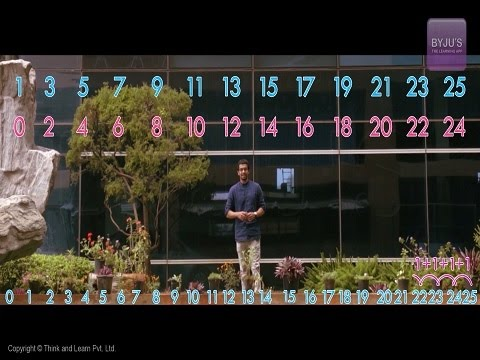### Number Pattern Example

Consider an example given here. The given sequence of numbers is 11, 17, 23, 29, 35, 41, 47, and 53. The following figure helps to understand the relationship between the numbers.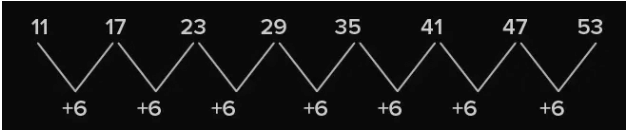In the given pattern, the sequence is increased by 6. It means the addition of the number 6 to the previous number gives the succeeding number. Also, the difference between the two consecutive number is 6.

## Number Patterns Using Dots

Let’s start representing each whole number with a set of dots and arranging these dots in some elementary shape to find number patterns. For arranging these dots, we take strictly four shapes into account. Numbers can be arranged into:

1. A line
2. A rectangle
3. A square
4. A triangle

## Line

Every number can be arranged in a line. Examples:

The number 2 can be represented by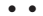The number 3 can be represented by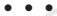All other numbers can be represented in a similar pattern.

## Rectangle

Some numbers can be arranged as a rectangle. Examples:

The number 6 can be arranged as a rectangle with 2 rows and 3 columns as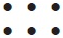Similarly, 12 can be arranged as a rectangle with 3 rows and 4 columns as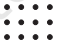Or as a rectangle with 2 rows and 6 columns as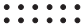Similar it can be formed by 8, 10, 14, 15, etc.

## Square

Some numbers can be arranged as squares. Examples:

The number 4 can be represented as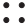and 9 as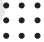Similar it can be formed by 16, 25, 36, 49 and so on.

## Triangle

Some numbers can be arranged as triangles. Examples:

The number 3 can be represented as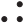and 6 as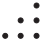Similar it can be formed by 10, 15, 21, 28, etc. It is to be noted that the triangle should have its 2 sides equal. Hence, the number of dots in the rows starting from the bottom row should be like 4,3,2,1. The top row should always have one dot.

## Number Pattern Types

There are different types of number patterns in Mathematics. They are:

• Arithmetic Sequence
• Geometric Sequence
• Square Numbers
• Cube Numbers
• Triangular Numbers
• Fibonacci Numbers

### Number Patterns Observation

Observation of number patterns can guide to simple processes and make the calculations easier.

Consider the following examples which help the addition and subtraction with numbers like 9, 99, 999, etc. simpler.

• 145 + 9 = 145 + 10 – 1 = 155 – 1 = 154
• 145 – 9 = 145 – 10 + 1 = 135 + 1 =  136
• 145 + 99 = 145 + 100 – 1 = 245 – 1 = 244
• 145 – 99 = 145 – 100 + 1 = 45 + 1 = 46

Consider another pattern which simplifies multiplication with 9, 99, 999, and so on:

• 62 x 9 = 62 x (10 – 1)  = 558
• 62 x 99 = 62 x (100 – 1)  = 6138
• 62 x 999 = 62 x (1000-1)  = 61938

Consider the following pattern which simplifies multiplication with numbers like 5, 25, 15, etc.:

• 48 x 5 = 48 x 10/2 = 480/2 = 240
• 48 x 25 = 48 x 100/4 = 4800/4 = 1200
• 48 x 125 = 48 x 1000/8 = 48000/8 = 6000

A number of patterns of similar fashion can be observed in the whole numbers which simplifies calculations to quite an extent.